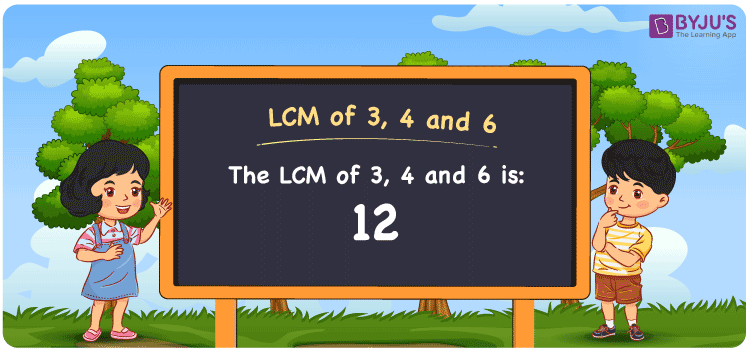Checkout JEE MAINS 2022 Question Paper Analysis : Checkout JEE MAINS 2022 Question Paper Analysis :

# LCM of 3, 4 and 6

LCM of 3, 4 and 6 is 12. Students can learn how the least common multiple of 3, 4 and 6 can be obtained by considering the common multiples. (3, 6, 9, 12, 15, 18, ….), (4, 8, 12, 16, 20, …..) and (6, 12, 18, 24, 30, 36,….) are the multiples of 3, 4 and 6. The LCM of two numbers can be calculated using methods such as prime factorisation, by listing the multiples and division.

## What is LCM of 3, 4 and 6?

The answer to this question is 12. The LCM of 3, 4 and 6 using various methods is shown in this article for your reference. The LCM of two non-zero integers, 3, 4 and 6, is the smallest positive integer 12 which is divisible by both 3, 4 and 6 with no remainder.## How to Find LCM of 3, 4 and 6?

LCM of 3, 4 and 6 can be found using three methods:

• Prime Factorisation
• Division method
• Listing the multiples

### LCM of 3, 4 and 6 Using Prime Factorisation Method

The prime factorisation of 3, 4 and 6, respectively, is given by:

3 = 3 = 3¹

4 = 2 x 2 = 2²

6 = 2 x 3 = 2¹ x 3¹

LCM (3, 4, 6) = 12

### LCM of 3, 4 and 6 Using Division Method

We’ll divide the numbers (3, 4, 6) by their prime factors to get the LCM of 3, 4 and 6 using the division method (preferably common). The LCM of 3, 4 and 6 is calculated by multiplying these divisors.

 2 3 4 6 2 3 2 3 3 3 1 3 x 1 1 1

No further division can be done.

Hence, LCM (3, 4, 6) = 12

### LCM of 3, 4 and 6 Using Listing the Multiples

To calculate the LCM of 3, 4 and 6 by listing out the common multiples, list the multiples as shown below

 Multiples of 3 Multiples of 4 Multiples of 6 3 4 6 6 8 12 9 12 18 12 16 24 15 20 30 18 24 36

LCM (3, 4, 6) = 12

## Video Lesson on Applications of LCM## LCM of 3, 4 and 6 Solved Examples

What is the smallest number exactly divisible by 3, 4 and 6.

Solution:

We know that

The smallest number exactly divisible by 3, 4 and 6 is the LCM.

Multiples of 3 = 3, 6, 9, 12, 15, 18,…..

Multiples of 4 = 4, 8, 12, 16, 20, 24, ….

Multiples of 6 = 6, 12, 18, 24, 30, 36, ….

Hence, the LCM of 3, 4 and 6 is 12.

## Frequently Asked Questions on LCM of 3, 4 and 6

### In the numbers 3, 5, 7, 12, what is the LCM of 3, 4 and 6?

The smallest multiple which is common and evenly divisible by 3, 4 and 6 gives the LCM. The number 12 satisfies this condition.

### List the methods used to determine the LCM of 3, 4 and 6.

The methods used to determine the LCM of 3, 4 and 6 are

Prime factorisation

Division

Listing the multiples

### Mention the relation between GCF and LCM of 3, 4 and 6.

The relation between GCF and LCM of 3, 4 and 6 is

LCM x GCF = 3 x 4 x 6

LCM x GCF = 72